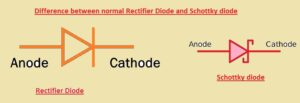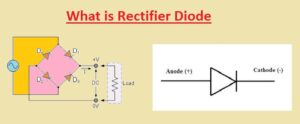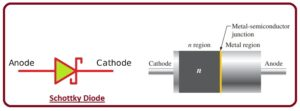Hello, readers welcome to the new post. Here we will learn Difference between a normal Rectifier Diode and Schottky diode. The diode is an electronic component that is used to flow current in one direction and not allow current in the opposite direction which means it transforms ac to DC current.

There are different types of diode used in electronics like LED, photodiode, Schottky diode, etc. In this post, we will discuss he differences between the Schottky diode and the rectifier diode. So let’s get started Difference between a normal Rectifier Diode and Schottky diode

### Introduction to Diode

• A Rectifier diode is an electronic component that converts alternating current to direct current through a rectification process and allows current to flow in one direction only.
• The operation of a diode is similar to that of a non-return valve, which allows liquid to flow in one direction only, similarly current flows through the diode in only one direction.
• The diode is made of a combination of two different semiconductor materials P and N.
• The P side of the semiconductor is positive and is called the anode, and the N side of the diode is negative and is called the anode.
• Because of the high-temperature bears, silicon is used in diode construction, but germanium is also used to make diodes when less voltage drop is needed.#### Diode Forward Biasing

• Biasing is the process by which the input power is secured across the diode terminals.
In the biased state, current flows through the PN junction due to the movement of electrons and holes.
In the given figure, you can see that the diode is connected to a DC voltage source in the forward direction.,
• The bias voltage supplied by the external DC source is represented as Vbias.
You can see that in the circuit a resistor is connected in series with the battery and the diode, this is to limit the current value and is known as a current-limiting resistor.
For the bias condition, 2 conditions should be met.
• The first is to connect the anode of the diode to the positive pole of the battery and the cathode to the negative pole of the battery.
• In the second condition, the value of the voltage source connected to the diode should be greater than the potential barrier.### Introduction to Schottky Diode

• Schottky diodes are also called hot carrier diodes or Schottky barrier diodes used in such applications where large frequency and high switching speed are required.
It was called a hot carrier diode because of the high energy level of the electrons in the N part than in the metal part of the diode.
• The diode symbol is shown in the figure below. To construct this normally N-doped diode semiconductor material, the material is combined with metals such as silver, gold, or platinum.
There is no PN junction in this diode like other diodes but it has a semiconductor-to-metal junction.
The forward voltage loss for this diode is normally 0.3 volts due to the absence of depletion of this diode.
The current flow of this diode is due to majority charge carriers and there are no minority charge carriers and also no reverse leakage current flow in this diode.
• The metal part of this diode is completely covered by conduction band electrons and the n region of this diode is less doped.
When the diode is forward-biased, the high-energy electrons existing in the N part move to the metal part and lose their energy in that region.
Due to the absence of minority charge carriers in this diode, the bias voltage changes rapidly.
This diode is used in higher frequency and switching applications such as digital circuits.#### Schottky Diode Construction

• In a Schottky diode, a metal-semiconductor junction is formed between the metal material and the semiconductor, which is called the Schottky barrier.
The construction of this diode uses gold, platinum, tungsten, and some silicides with a semiconductor material that is N-doped.
The metal part of this diode acts as the anode and the N part is the cathode, which means that the current can move from the metal part to the semiconductor according to the conventional current direction.
The Schottky barrier of this diode ensures high-speed switching and less forward bias loss.
The forward voltage required for a diode depends on the metal and semiconductor material used. Both N-type and P-type semiconductor materials can be used to make the Schottky barrier, but the forward bias for the P-type material is smaller.
As the forward bias voltage decreases, the reverse leakage current increases so that the voltage value for the P-type material is maintained in the range of 0.5 to 0.7 volts.
Parameter Normal Rectifier Diode Schottky Diode
Voltage Drop High Low
Reverse Recovery Time High Low
Forward Voltage Drop High Low
Efficiency Low High
Applications Power Supplies, Battery Chargers, and AC-DC Converters Power Supplies, Voltage Clamping, and RF Applications